## Homework 7a - Ghost

This project is a neat, algorithmic problem that uses Python on masses of data. There is also a smaller HW7b program. All parts of HW7 are due Wed Nov 17 at 11:55pm. Download ghost.zip to get started.

## Warmups

We have a few warmups functions to get ready for the Ghost problem. These all have a 1-line solution using lambda.

Turn In Turn In Warmups to Paperless

## Ghost Problems

Suppose we are trying to take a picture of Stanford, but each image has people walking through the scene, like this (this is the "hoover" data set):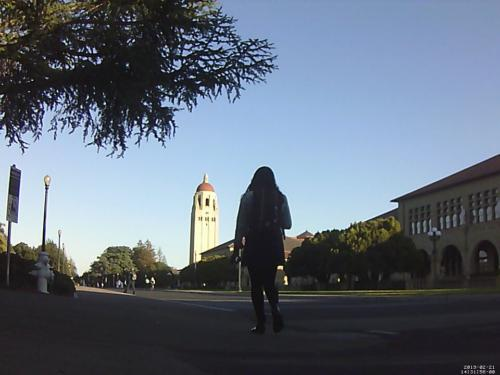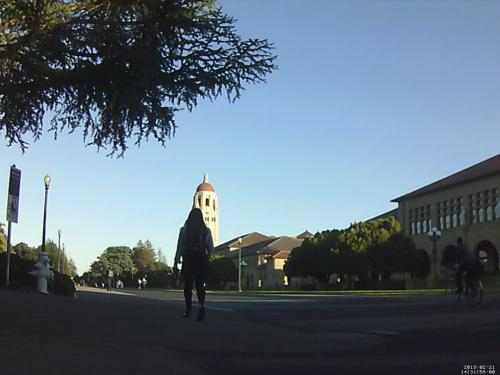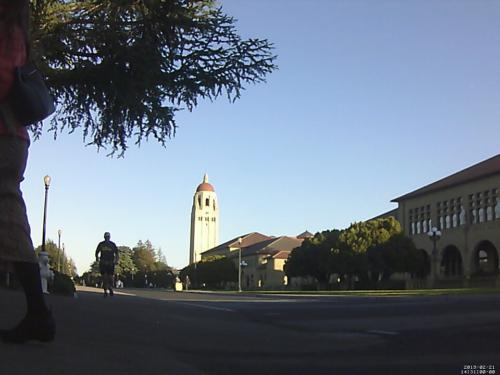We'd like to look through all these images and figure out an image that "ghosts" out all the people and just shows the background. (Thanks to John Nicholson for his "Pesky Tourist" Nifty Assignment which inspired this project, see nifty.stanford.edu).

## Ghost Solution

This assignment depends on the "Pillow" module you installed for an earlier. Instructions for installing Pillow are included at the end of this document if necessary.

Programming detail: For this assignment, we'll access the color of a pixel as a "pix" - a len-3 tuple, e.g. (red, green, blue) as shown below. The SimpleImage module supports getting and setting pix data on images with get_pix() and set_pix() functions.

Say we have 4 images. For every x,y consider the 4 pixels at that x,y across all the images. Many of those pixels show the ordinary background, and a few pixels have someone walking in front, which we'll call "outlier" pixels. We'll assume that outlier pixels are not the majority for any x,y.

With 4 images, suppose the 4 pix at x=100 y=50 look like:

```[(1, 1, 1), (1, 1, 1), (28, 28, 28), (1, 1, 1)]
```Looking at the pixs, you can guess that (28, 28, 28) is the outlier and (1, 1, 1) is the background since it's the majority. What's an algorithm to figure out the true background pixel for an x,y?

## Color Distance

To frame this problem, it's handy to introduce the idea of "color distance" between pix. You can think of each pix color as a point in a 3-dimensional color space, with red-green-blue coordinates instead of the regular x-y-z coordinates.

For reference, the standard formula for distance in x-y-z space is (in Python syntax):

```math.sqrt(x_difference ** 2 + y_difference ** 2 + z_difference ** 2)
```

Reminder: In Python "**" is exponentiation, so (x ** 2) is x squared. The module named "math" contains miscellaneous math functions, and math.sqrt() is the square root function. As a surprising optimization, we can actually omit the math.sqrt() for this algorithm (explained below).

## Ghost Algorithm

There are a few ways to solve the Ghost problem, but the following is a relatively simple approach that works well.

Problem: for any particular x,y, look at all the pix across the images at that x,y. We want to pick out the best pix to use, avoiding the outlier pix.

1. Compute the floating point arithmetic average pix across these pixs - average all the red values to get average-red-value, and likewise compute the average-green-value and average-blue-value. So for example, the three pix (1, 1, 1), (1, 1, 31), (28, 28, 28) would define an average of (10.0, 10.0, 20.0). It's fine to use a simple loop/+= to compute this.

To think through the algorithm, imagine the pixs for some x,y scattered in a 2D space of colors.All the pix but the outlier will naturally cluster, grouped around the theoretically perfect color for that x,y, but displaced by little measurement errors. The outlier pix will be off by itself, a truly different color. The average will be in between the two, but nearer to the cluster, since the cluster has many pix and the outlier has just one.

2. To select the best among the pixs, select the pix with the least distance to the average of all the pixs. Equivalently we could say the the pix closest to the average. This works because the more numerous background pixs are near the average, while any outlier pix are relatively far from the average.

## Sqrt Performance Trick

In order to select the closest pix, we can omit the math.sqrt(), which is great because square-root is a relatively slow math operation. Here's why: suppose we have three pix, and their distances from average are 4, 6, and 9. We would select the distance "4" one as the closest. However, that works the same if distances are squared, which would be 16, 36, and 81 - we select the "16" one. Computing the square root was unnecessary to selecting the one with the least distance. Therefore, in the code below, we'll compute the distance squared instead of the distance, and in fact using distance-squared is a standard big-data technique for just this reason.

## Ghost Code

Three functions are specified below and are stubbed out in the starter code. You will need to write some additional helper functions too.

## 1. pix_dist2(pix1, pix2)

Write the code for a pix_dist2() function which returns the distance-squared between 2 pix. Write at least 2 Doctests.

```def pix_dist2(pix1, pix2):
"""
Returns the square of the color distance between 2 pix tuples.
"""
```

This is a low-level function, whose role is to be called by other functions. Often in our programs, the low-level functions are first in the file, called by the functions later in the file.

## 2. best_pix(pixs)

Write the code for a best_pix() function which, given a list of pix, returns the best pix from that list according to the ghost algorithm. For example with the pixs [(1, 1, 1), (1, 1, 1), (28, 28, 28)] this should return (1, 1, 1). The number of pix will be at least 3, but may be more. Write at least 2 Doctests. One of the Doctests should have more than 3 pix in the input.

Design constraint: best_pix() should not itself loop through the pix to compare distances. Instead, it should leverage lambda to pick out the best pix.

Helper function: looking at the algorithm for best_pix() suggests the need for an average_pix() helper function - given a list of pix, compute an average pix where the red value is the average of all the red values, the green is the average of all the green, and so on. Nothing is provided in the starter code for this, so put it in yourself, including """Pydoc""" and at least 2 Doctests. This is the "top-down" decomposition direction, where working on some function, you think up a helper that would be handy.

```def best_pix(pixs):
"""
Given a list of 3 or more pix, returns the best pix.
"""
```

If multiple pix qualify as the best, the code is free to pick any one of them.

## Testing Strategy

Looking at the the whole ghost program output for, say, the hoover case, it's hard to tell for sure if the program is working correctly. In contrast, best_pix() has been made testable by stripping it down to the minimum - a list of pixs go in, one pix comes out. That form is so simple, it can be tested and made correct. Once best_pix() is correct, the program routes this river of data through that one, well-tested function.

Cultural aside: this Sesame Street song is weirdly reminiscent of the Ghost algorithm.

## 3. solve(images)

This is a high level function, solving the whole problem, but calling other functions to solve sub-problems. The provided main() does the housekeeping of loading the image objects, and then calls solve() to do the real work. Solve() does not need Doctests.

```def solve(images):
"""
Given a list of image objects, compute and show
a Ghost solution image based on these images.
There will be at least 3 images and they will all be
the same size.
"""
```

Create a solution image the same size as the first image in the list. After the all the pix are set in the solution, call the show() function to put it on screen.

```    solution = SimpleImage.blank(width, height)
...
# do all the work
...
solution.show()
```

Helper function: decompose out a pixs_at_xy(images, x, y) helper function that takes in the list of images and an x,y, returns a list of all the pix at that x,y. This is a sub-part of solve(), but it makes a nice helper function. This function is not in the starter code, so create it with """Pydoc""" but it does not need tests.

## SimpleImage Functions

The provided SimpleImage module has two functions that access image data in the "pix" format of (red, green, blue) tuples. The old get_pixel() function works too, but for this project, do everything in "pix" - get_pix() and set_pix().

```SimpleImage Features:
Create image:
image = SimpleImage.blank(400, 200)   # create new image of size
image = SimpleImage('foo.jpg')        # create from file

Access size
image.width, image.height
image.in_bounds(x, y)      # boolean test

Get pix at x,y
pix = image.get_pix(x, y)
# pix is RGB tuple like (100, 200, 0)

Set pix at x,y
image.set_pix(x, y, pix)   # set data by tuple also

Get Pixel object at x,y
pixel = image.get_pixel(x, y)
pixel.red = 0
pixel.blue = 255

Show image on screen
image.show()
```

## Ghost Run

The ghost.py program takes just one command line argument - the name of the directory that contains the jpg files. The folder "hoover" is relatively small, containing the three jpg files (the three example images at the top of this page). Run the program with the "hoover" files like this:

```\$ python3 ghost.py hoover
hoover/200-500.jpg
hoover/158-500.jpg
hoover/156-500.jpg
(solution image appears)
```

The provided main() first looks in the folder, loads all the jpg files within the folder, and prints the filename of each image as it goes. Then main() calls your solve() function, passing in the images to do the real work.

## Hoover Solution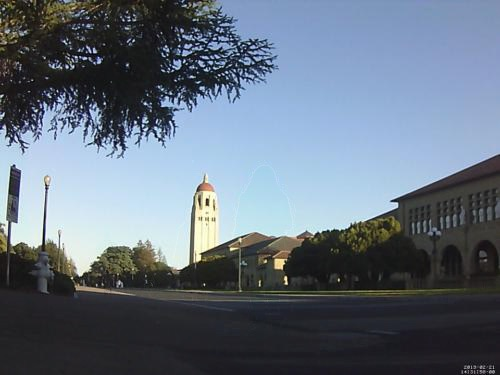## Bigger Test Cases

We have bigger and better campus cases to try, each in a folder: clock-tower, math-corner, and monster. For any of these, you can look at the individual images inside the folder to get a feel for the data. The file naming convention here is that the file "foo-500.jpg" is 500 pixels wide.

### Clock Tower

Here's a medium sized case by the clock tower.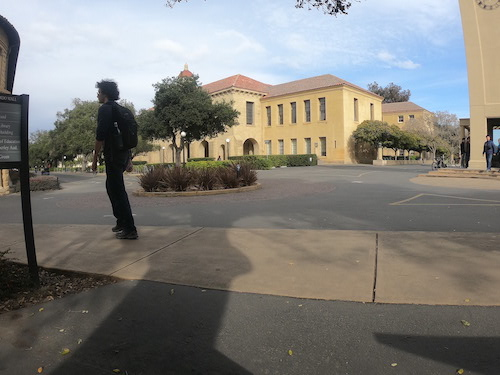```\$ python3 ghost.py clock-tower
```

### Math Corner

A larger case by math corner.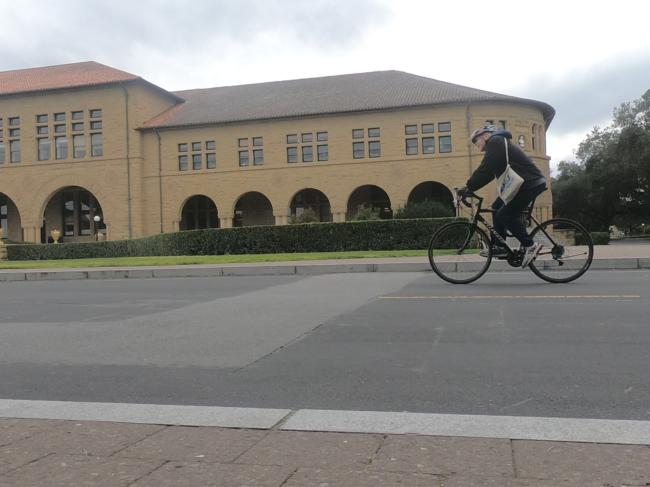```\$ python3 ghost.py math-corner
```

### Monster

And finally this "monster" case - eight large images again in the direction of of Hoover tower. If you look really carefully, you may see a faint shadow in the output, marking where outliers have been removed. This is fine, just hitting the limit of what this algorithm can do.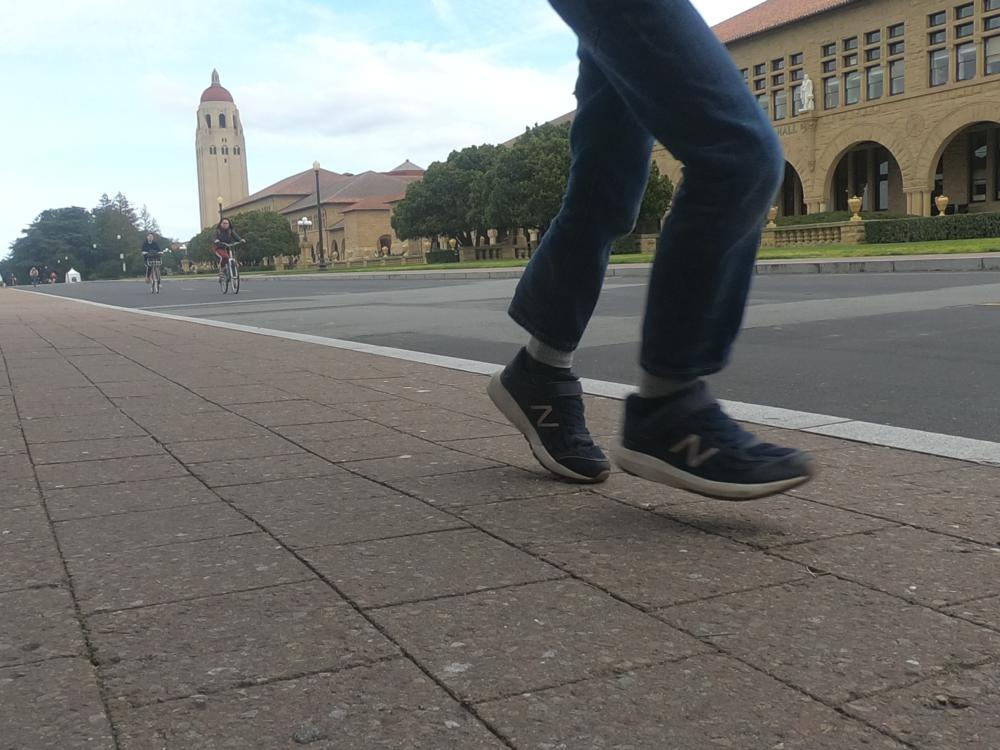```\$ python3 ghost.py monster
```

The monster images are each 1000 x 750 and there are 8 image files, and each pixel has 3 numbers. How many numbers is that?

```>>> 1000 * 750 * 8 * 3
18000000
```

Your code is sifting through 18 million numbers to figure out the ghost image.

When your code is cleaned up and works correctly, that's a dense algorithmic core with very visual output. Please turn in your ghost.py on Paperless as usual.

## Appendix: Installing Pillow

This project will use the "Pillow" library. The Pillow library may already be installed on your machine from an earlier homework. The steps below will install Pillow if needed.

Open a "terminal" window - the same type of window where you type "python3 foo.py" to run programs. The easiest way to get a Terminal is open the Ghost project in PyCharm, and use the "terminal" tab to the lower left. Type the following command (shown in bold as usual). Note that "Pillow" starts with an uppercase P. (On windows, "py" instead of "python3").

```\$ python3 -m pip install Pillow
..prints stuff...
Successfully installed Pillow-5.4.1
```

To test that Pillow is working, type the following command to a terminal inside your homework folder. This runs the "simpleimage.py" code included in the folder. When run like this, simpleimage.py creates and displays a big yellow rectangle with green at the right edge.

```# (inside your project folder)
\$ python3 simpleimage.py
# yellow rectangle appears
```